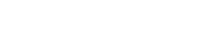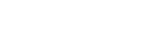# Using Q-Learning for Pathfinding

Serengeti
18.06.2019.## Introduction

Reinforcement learning is a part of machine learning that deals with finding the best action to take in a given situation. Unlike supervised learning where the training data is already classified, in reinforcement learning agent does not know if the actions it is about to take are correct or not. Agent must take the action and observe its consequences to determine the value of that action. Consequences of an action is a reward that the agent receives. By rewarding or punishing an agent for actions it takes, we are reinforcing the desired behavior. Hence the name reinforced learning.

One popular algorithm of reinforcement learning is Q-learning. Purpose of Q-learning algorithm is to learn a quality function that will give us correct action for a given state. Q-learning has a simple formula:

Q[state, action] = R(state, action) + Gamma * Max[Q(next state, all actions)]

Q is a quality function that for a given state and an action returns a value of that state-action pair. In supervised learning that value is known. In reinforced learning our agent must learn this function. R is a reward function that we use to reinforce agent behavior by rewarding or punishing its choices. Gamma is a number between 0 and 1 that determines will the agent value more immediate reward provided by R function or a delayed reward provided by Q function.

## Q-learning

We are going to use a simple logic puzzle to show how q-algorithm works. It is a form of a “river crossing” puzzle. On the left side of the river we have two missionaries, two cannibals and a single boat with maximum capacity of three persons. We must transfer all four persons to the right side of the river given these constraints:

1. Persons can cross the river only in the boat.
2. At least one person must be in the boat during the crossing of the river.
3. Maximum number of persons that can be in the boat is three.
4. If cannibals outnumber missionaries on either side of the river in any moment, they will eat those missionaries and we will not be able to solve the puzzle.

We can represent initial state as [MMCC<---]  where letter M represents a single missionary, letter C a single cannibal and arrow shows on which side of the river the boat is.

Goal state is therefore represented as [--->MMCC] with both, cannibals and missionaries on the right side of the river.

We want our agent to learn a sequence of action that will take it from initial state to the goal state. For our agent to learn we must reward him for desired behavior. In this case that is reaching the goal state. Therefore, we will reward our agent for taking an action that leads it to goal state. If an action is leading back to initial state, we will discourage our agent with negative reward. We will give no reward for any other action.

We can represent states, actions, Q and R functions in a form of a directed graph. States will be nodes, actions will be edges that connect those nodes and Q and R function will be values assigned to those nodes. Only those edges that lead to goal state will have R value of 1. Edges that lead to initial state will have R value of -1. All other edges will have a R value of 0. We do not know yet what is the value of Q function so we will assign Q value of 0 to every edge. We can think of q-algorithm as a process of transforming unweighted directed graph into a weighted directed graph.

We will set Gamma value to 0.6 because we reward our agent only when it reaches goal state so we want it to value delayed reward. We will run 100 iterations of learning. Each starting in a known node and ending once the goal node is reached. Each iteration will update the Q function and Q values in their corresponding edges of the graph.

Algorithm for learning Q function is:

1. Set Gamma and R values for known nodes
2. Initialize Q values to 0 for known edges
3. For each iteration of learning:
1. Select one known node as current node
2. While not in goal node:
1. Randomly select one possible neighboring node as next node
2. Get R value of edge leading from current node to next node
3. Get maximum Q value of all edges leaving this next node
4. Compute and update Q (current nodenext node) = R(current nodenext node) + Gamma * Max[Q(next node, all edges from next node)]
5. Set next node as current node

Once we run all learning iterations, we should have optimized Q values and we can use those values with this algorithm:

1. Set current node to initial node
2. While current node is not goal node
1. Select an edge from current node that has highest Q value
2. Follow that edge to new node and set that new node as current node

## Example

Step 1

We start first iteration of learning in start node [CCMM<---]. Our agent uses four puzzle rules to determine neighboring nodes/states. Since none of the neighboring nodes is an initial or goal node we set R values for all four edges to 0. We also set Q values for all edges to 0 because this is the first time we have seen those edges.

We randomly select [MMC--->C] as next node and explore it. We find out that only edge leading from that node is the one back to initial node. This is why we give that edge R value of -1. We give that edge Q value of 0 since it is a new edge. We calculate Q([MMCC<---], [MMC--->C]) = 0 + 0.6 * 0 = 0 which is the same as current Q value of that edge.

Step 2

We are now in node [MMC--->C] and we can go only to initial node [MMCC<---]. This time we calculate Q([MMC--->C], [MMCC<---]) = -1 + 0.6 * 0 = -1 and update that Q value. This is the first knowledge we have gained so far. Our Q function just became a bit more accurate.

Step 3

We are back in initial node. This time we randomly choose edge leading to node [MC--->MC]. By exploring it we discover a new node [MMC<---C] and two new transitions. We assign R and Q values to newly discovered edges but we receive no new knowledge from this action.

Step 4

We are now in node [MC--->MC]. We randomly choose to go to node [MMC<---C] and explore it. We discover four new edges and two new nodes. One of them being goal state. We assign R and Q values and go to new node.

Step 5

From [MMC<---C] we randomly choose an edge that takes us to the goal node [--->MMCC]. We explore it and discover five new edges leading out of the goal node. We calculate Q([MMC<---C], [--->MMCC]) = 1 + 0.6 * 0 = 1 and update our Q function with new value.

Since we are in the goal node, this learning iteration is done. Our agent discovered new nodes and edges and has optimized his Q function. Later iterations will discover more nodes and edges and most importantly it will optimize Q function.

After 100 iterations, our agent has discovered 12 nodes and 29 edges. When using now optimized Q function to find shortest path from initial node to the goal node, we get this sequence.

1. [MMCC<---]
2. [MC--->MC]
3. [MMC<---]
4. [--->MMCC]

## Summary

Whether we are trying to solve a simple puzzle or trying to teach a robot to behave and explore an unknown environment, principles of reinforcement learning stay the same. Applications of reinforcement learning will continue to grow in numbers as robotics is becoming more common in our lives. I hope that this post gave you enough information to go and experiment with your own problems and applications.